# MA2051 - Ordinary Differential EquationsReview For Test II

1. Work with the following differential equation: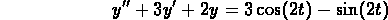(a)
Find two linearly independent solutions for the corresponding homogeneous equation. Prove that your solutions really are linearly independent.

(b)
Find a particular solutionfor the original equation.

(c)
Find the solution for the equation which satisfies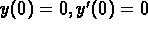.

(d)
Does the solution have a transient component? If it does, estimate how long it takes for the magnitude of this component to become less than.

(e)
Does the equation have a limiting periodic solution? If it does, identify the amplitude and period of this limiting solution.

(f)
Determine initial values (and) so that the solution to the initial value problem contains no transient component.

2. Consider the two functions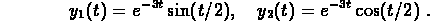(a)
Find a linear, constant-coefficient, homogeneous differential equation that has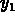and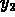as solutions.
(b)
Determine initial conditionsand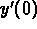so that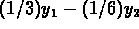is the solution for the differential equation that you found in (a).

3. A mass of 6 kg is suspended from a spring that extendedm when a 1 kg mass was attached. Assume that friction damping can be ignored (until part (d)). You pull the mass down5 cm and release it from rest.

(a)
Write down the mathematical model for this system. Use the given data to specify the numerical values for the parameters in the model.
(b)
Determine the natural frequency,the period,and the amplitude of the motion of the mass.
(c)
How much would you have to increase the mass in order to double the period? How much would you have to increase the mass in order to double the the amplitude?
(d)
Take the same spring-mass system and immerse it in a vat of molasses. It is no longer reasonable to ignore the force of friction damping. For what values of the damping coefficient p will the spring still exhibit oscillations about the steady state? Explain your answer.

4. Consider the following system of first-order differential equations: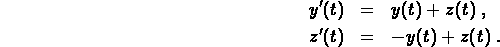(a)
Find two solution pairs and verify that they are linearly independent. Give the general solution for the system.
(b)
Find the solution satisfying initial conditionsand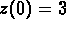.
(c)
Graph the solution obtained in part (b) in the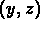--plane, you would find that it is a spiral going out away from the origin.
(d)
Using only the differential equation (and not the actual solution), show that the quantity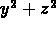is actually increasing along any solution curve and so the solution must be heading away from the origin.

5. Use two steps of Euler's method to approximate the solution toat t = 1. Compare your numerical result with the exact solution obtained in problem #1 above. What is the error?

© 1996 by
Will Brother. All rights Reserved. File last modified on April 25, 1996.